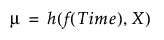Reliability and Survival Methods > Destructive Degradation > Statistical Details for the Destructive Degradation Platform
Publication date: 05/24/2021

# Statistical Details for the Destructive Degradation Platform

The destructive degradation model can be expressed as follows:where:

g(Y) is the transformed Y variable

F is the selected probability distribution

μ is the location parameter, defined by h

h is a function that relates the transformed Time variable and the explanatory variable X

σ is the scale parameter of the distribution

f(Time) is the transformed Time variable

X is an optional explanatory variable

The standardized residuals are obtained as follows:

For location-scale distributions, the standardized residuals are (y-μ)/σ.

For log-location-scale distributions, the standardized residuals are (log(y)-μ)/σ.